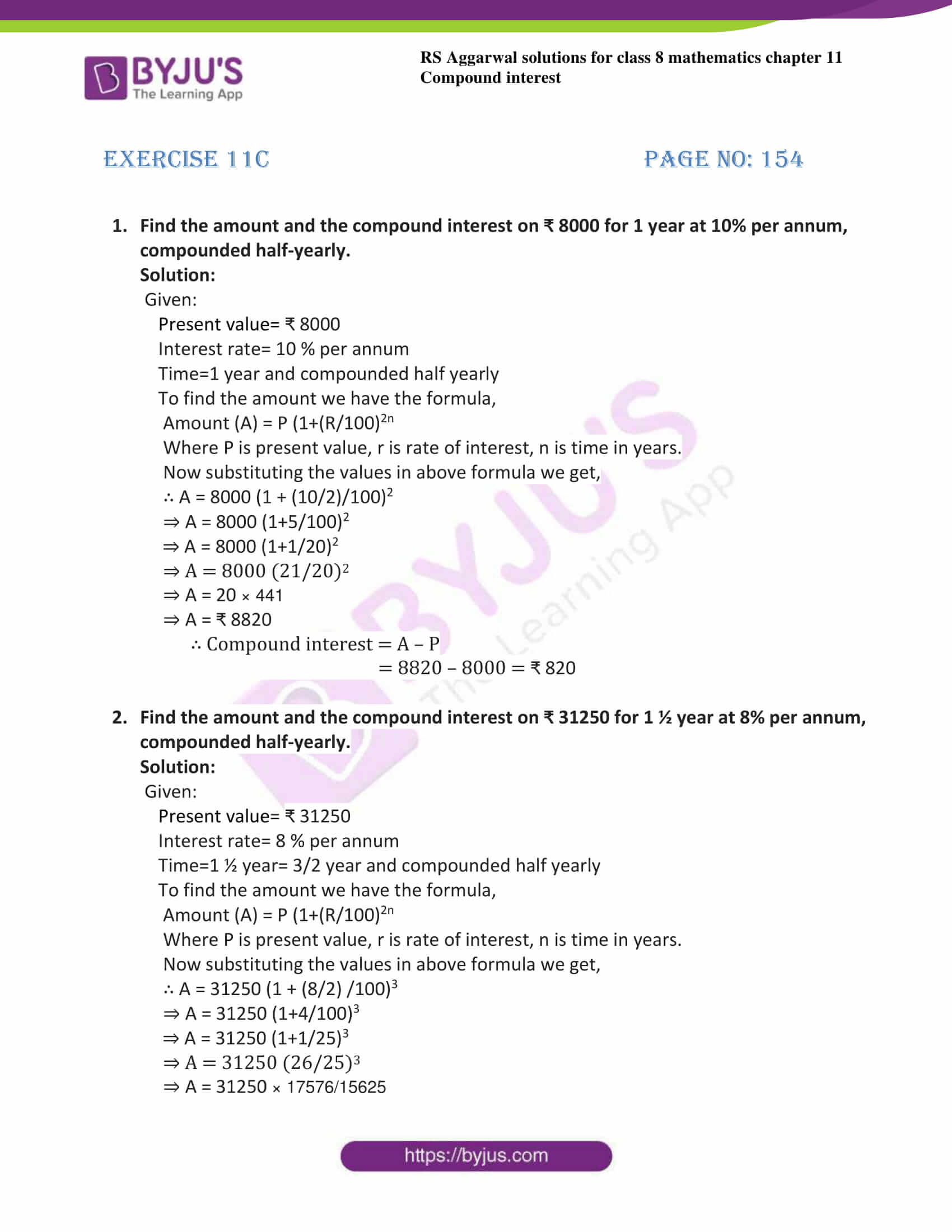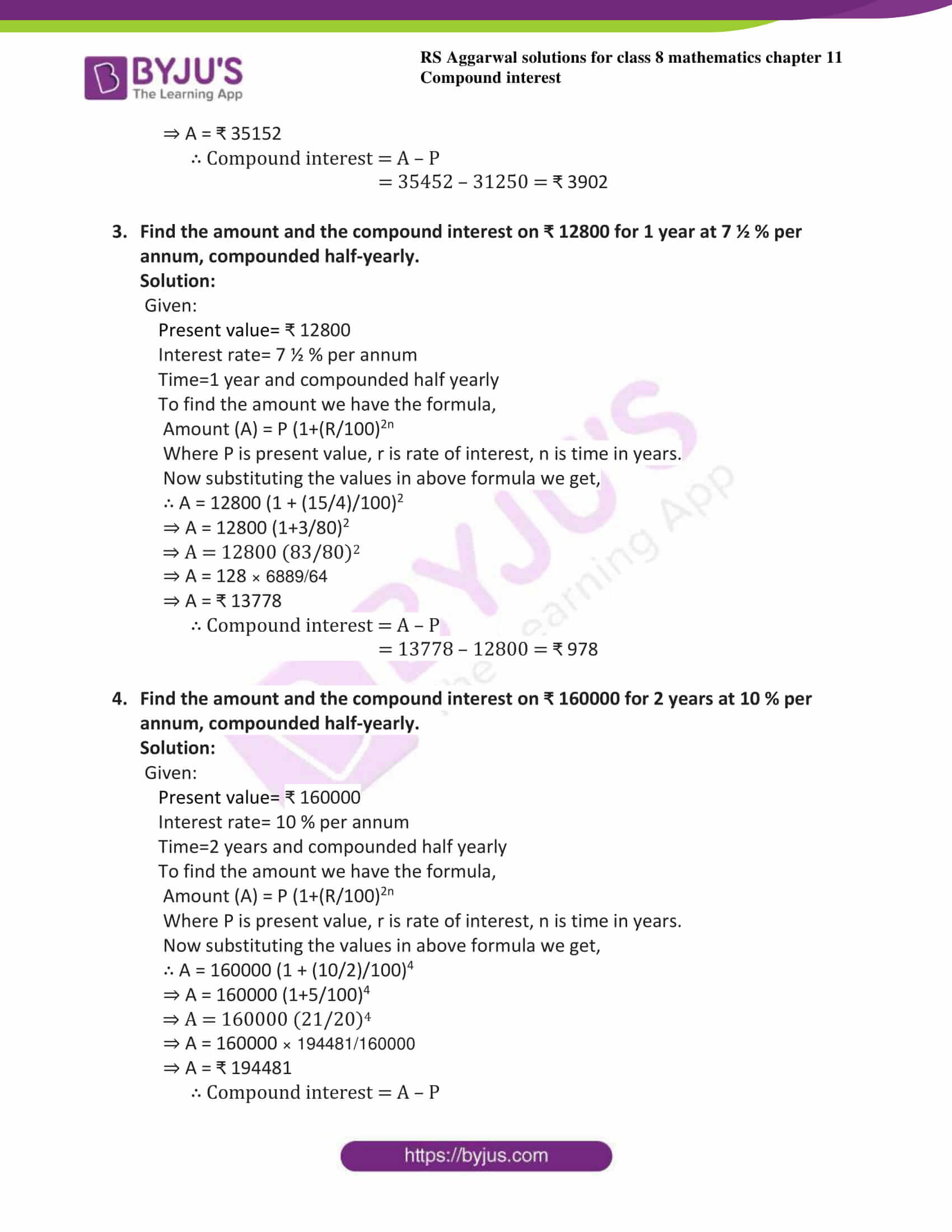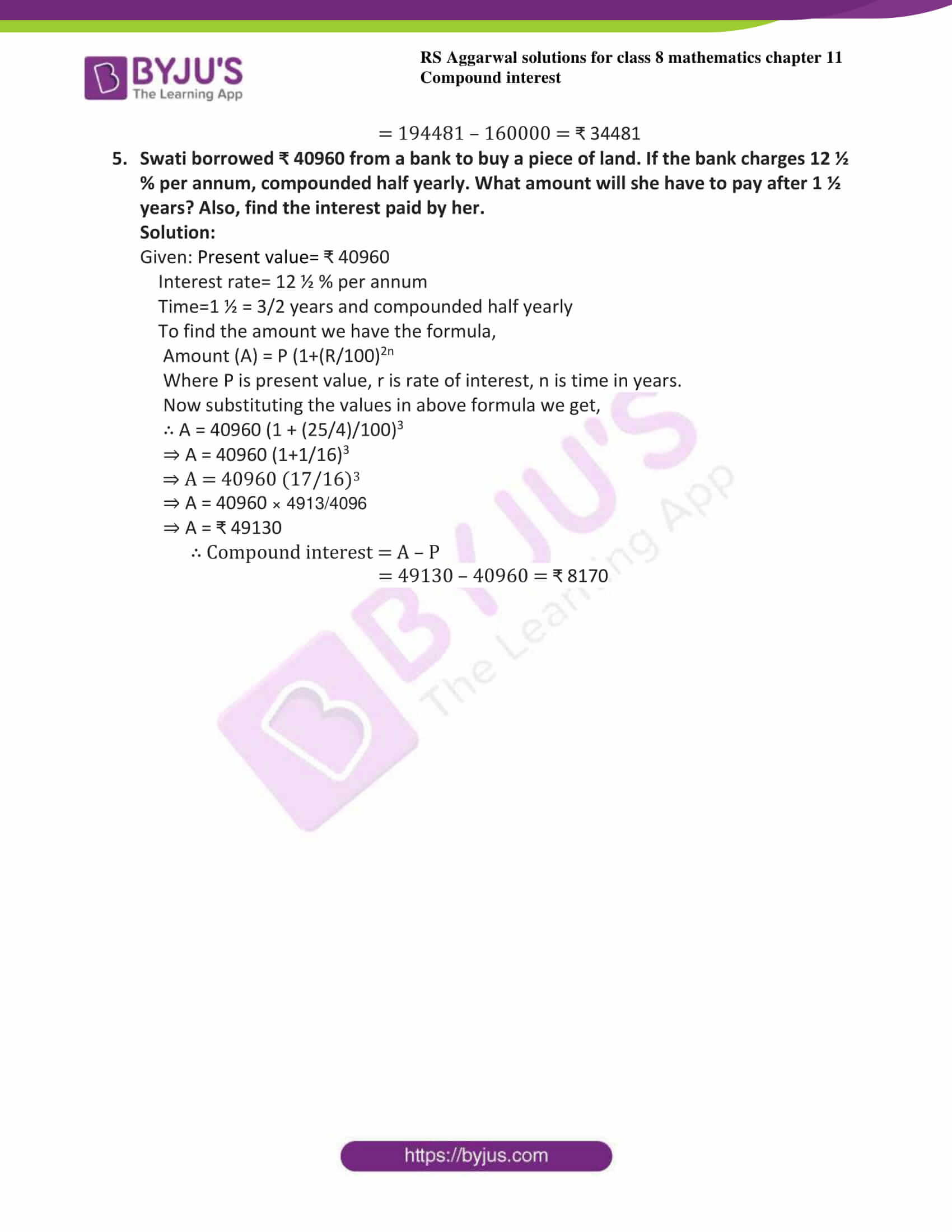# RS Aggarwal Solutions for Class 8 Maths Chapter 11 - Compound Interest Exercise 11C

RS Aggarwal Solutions for the Exercise 11C of Class 8 Maths Chapter 11, Compound Interest is provided here. BYJU’S expert team have solved in such a way that learners can understand easily and comfortably. This exercise includes ten questions based on finding the compound interest quarterly and half-yearly using the formulae. By exercising the RS Aggarwal Solutions for class 8, students will be able to grasp the concepts entirely. And, to acquire good scores, they have to go through RS Aggarwal Solutions are given here.

## Download PDF of RS Aggarwal Solutions for Class 8 Maths Chapter 11 – Compound Interest Exercise 11C### Access answers to Maths RS Aggarwal Solutions for Class 8 Chapter 11 – Compound Interest Exercise 11C

1. Find the amount and the compound interest on ₹ 8000 for 1 year at 10% per annum, compounded half-yearly.

Solution:

Given:

Present value= ₹ 8000

Interest rate= 10 % per annum

Time=1 year and compounded half yearly

To find the amount we have the formula,

Amount (A) = P (1+(R/100)2n

Where P is present value, r is rate of interest, n is time in years.

Now substituting the values in above formula we get,

∴ A = 8000 (1 + (10/2)/100)2

⇒ A = 8000 (1+5/100)2

⇒ A = 8000 (1+1/20)2

⇒ A = 8000 (21/20)2

⇒ A = 20 × 441

⇒ A = ₹ 8820

∴ Compound interest = A – P

= 8820 – 8000 = ₹ 820

2. Find the amount and the compound interest on ₹ 31250 for 1 ½ year at 8% per annum, compounded half-yearly.

Solution:

Given:

Present value= ₹ 31250

Interest rate= 8 % per annum

Time=1 ½ year= 3/2 year and compounded half yearly

To find the amount we have the formula,

Amount (A) = P (1+(R/100)2n

Where P is present value, r is rate of interest, n is time in years.

Now substituting the values in above formula we get,

∴ A = 31250 (1 + (8/2) /100)3

⇒ A = 31250 (1+4/100)3

⇒ A = 31250 (1+1/25)3

⇒ A = 31250 (26/25)3

⇒ A = 31250 × 17576/15625

⇒ A = ₹ 35152

∴ Compound interest = A – P

= 35452 – 31250 = ₹ 3902

3. Find the amount and the compound interest on ₹ 12800 for 1 year at 7 ½ % per annum, compounded half-yearly.

Solution:

Given:

Present value= ₹ 12800

Interest rate= 7 ½ % per annum

Time=1 year and compounded half yearly

To find the amount we have the formula,

Amount (A) = P (1+(R/100)2n

Where P is present value, r is rate of interest, n is time in years.

Now substituting the values in above formula we get,

∴ A = 12800 (1 + (15/4)/100)2

⇒ A = 12800 (1+3/80)2

⇒ A = 12800 (83/80)2

⇒ A = 128 × 6889/64

⇒ A = ₹ 13778

∴ Compound interest = A – P

= 13778 – 12800 = ₹ 978

4. Find the amount and the compound interest on ₹ 160000 for 2 years at 10 % per annum, compounded half-yearly.

Solution:

Given:

Present value= ₹ 160000

Interest rate= 10 % per annum

Time=2 years and compounded half yearly

To find the amount we have the formula,

Amount (A) = P (1+(R/100)2n

Where P is present value, r is rate of interest, n is time in years.

Now substituting the values in above formula we get,

∴ A = 160000 (1 + (10/2)/100)4

⇒ A = 160000 (1+5/100)4

⇒ A = 160000 (21/20)4

⇒ A = 160000 × 194481/160000

⇒ A = ₹ 194481

∴ Compound interest = A – P

= 194481 – 160000 = ₹ 34481

5. Swati borrowed ₹ 40960 from a bank to buy a piece of land. If the bank charges 12 ½ % per annum, compounded half yearly. What amount will she have to pay after 1 ½ years? Also, find the interest paid by her.

Solution:

Given: Present value= ₹ 40960

Interest rate= 12 ½ % per annum

Time=1 ½ = 3/2 years and compounded half yearly

To find the amount we have the formula,

Amount (A) = P (1+(R/100)2n

Where P is present value, r is rate of interest, n is time in years.

Now substituting the values in above formula we get,

∴ A = 40960 (1 + (25/4)/100)3

⇒ A = 40960 (1+1/16)3

⇒ A = 40960 (17/16)3

⇒ A = 40960 × 4913/4096

⇒ A = ₹ 49130

∴ Compound interest = A – P

= 49130 – 40960 = ₹ 8170

## RS Aggarwal Solutions for Class 8 Maths Chapter 11 – Compound Interest Exercise 11C

Exercise 11C of RS Aggarwal Solutions for Chapter 11, Compound Interest covers all the topics related to compound interest and methods of solving compound interest using the formulae. Some of the topics focused prior to exercise 11C include the following.

• Definition of compound interest
• To find compound interest when interest is compounded annually
• To find compound interest when interest is compounded half-yearly
• Calculating the compound interest by using formulae
• Applications of compound interest formula

The RS Aggarwal Solutions can help the students in practising and learning each and every concept as it provides solutions to all questions asked in the RS Aggarwal textbook.